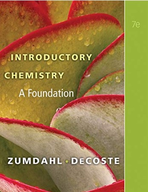Introductory Chemistry: A Foundation - 7 Edition - Chapter 13 - Problem 139
Register Now

Join StudySoup

Get Full Access to Introductory Chemistry: A Foundation - 7 Edition - Chapter 13 - Problem 1399781439049402

# A flask of hydrogen gas is collected at 1.023 atm and 35 C by displacement of water from

Introductory Chemistry: A Foundation | 7th Edition

Problem 139

A flask of hydrogen gas is collected at 1.023 atm and 35 C by displacement of water from the flask. The vapor pressure of water at 35 C is 42.2 mm Hg. What is the partial pressure of hydrogen gas in the flask?

Accepted Solution
Step-by-Step Solution:
Step 1 of 3

Chemistry 111 Lecture Notes (Dr. Patton) 3.8- Amounts of reactants and products  Stoichiometry  Definition: Qualitative study of reactants and products in a chemical reaction in a chemical reaction  EXAMPLE: 2 H (g) +2O (g) 2 2H 2(g) How many moles of water can be formed if… a) 1 mol of O r2acts completely with H 2 Begin with given (1 mol O ) 2 1 mol O (2 mol H O/1 mol O ) = 2 mol H O 2 2 2 2 b) 0.41 mole of O react completely with H 2 2 Begin with given (0.41 mol of O ) …2 0.41 mol O (2 mol H O/12mol O ) =.82 mol H O 2  A

###### Chapter 13, Problem 139 is Solved

Step 2 of 3

Step 3 of 3

Unlock Textbook Solution